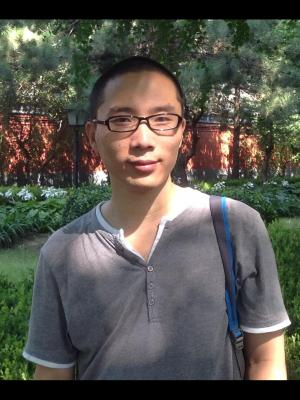Recent Developments in Finite Element Methods on Triangular Meshes for Convection-dominated Problems
Speaker
Dr. Jin Zhang
School of Mathematical Sciences, Shandong Normal University
Abstract

In this talk, I will present some recent developments in finite element methods on triangular meshes for convection-dominated problems. A series of integral inequalities on triangular meshes are derived, which are essential for estimates of diffusion and convection terms. Based on these inequalities, we analyze the superconvergence behavior of streamline diffusion finite element methods (SDFEMs), which reveals the differences between linear SDFEMs and bilinear ones. With the help of weighted estimates, these superconvergence results yield optimal order L² errors. Also pointwise errors can be obtained by bounds of discrete Green functions and careful analysis of the bilinear form. In the meantime, the above results provide a clue for choices of linear and bilinear elements in exponential layers and characteristic layers.